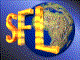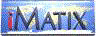| iMatix home page | << | < | > | >>SFLVersion 2.11

### timer_to_time

```#include "sfldate.h"
long
timer_to_time (time_t time_secs)
```

#### Synopsis

Converts the supplied timer value into a long time value. Times are stored as long values: HHMMSS00. Since the timer value does not hold centiseconds, these are set to zero. If the supplied value was zero, returns zero. The timer value is assumed to be UTC (GMT).

#### Source Code - (sfldate.c)

```{
struct tm
*time_struct;

if (time_secs == 0)
return (0);
else
{
/*  Convert into a long value HHMMSS00                               */
time_struct = safe localtime (&time_secs);
return (MAKE_TIME (time_struct-> tm_hour,
time_struct-> tm_min,
time_struct-> tm_sec,
0));
}
}
```

 | << | < | > | >>Copyright © 1996-2000 iMatix Corporation